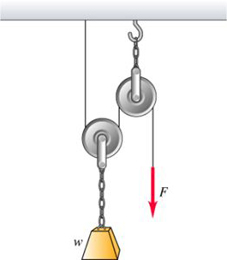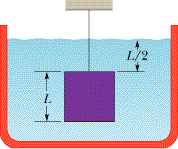Ask question

# In the figure a worker lifts a weight \omega by pulling down on a rope with a force \vec{F}. The upper pulley is attached to the ceiling by a chain,and the lower pulley is attached to the weight by another chain.The weight is lifted at constant speed. Assume that the rope,pulleys, and chains all have negligible weights. A) In terms of \omega,find the tension in the lower chain. B) In terms of \omega,find the tension in upper chain. C) In terms of \omega,find the magnitude of the force \vec{F} if the weight is lifted at constant speed.# In the figure a worker lifts a weight \omega by pulling down on a rope with a force \vec{F}. The upper pulley is attached to the ceiling by a chain,and the lower pulley is attached to the weight by another chain.The weight is lifted at constant speed. Assume that the rope,pulleys, and chains all have negligible weights. A) In terms of \omega,find the tension in the lower chain. B) In terms of \omega,find the tension in upper chain. C) In terms of \omega,find the magnitude of the force \vec{F} if the weight is lifted at constant speed.

Question
Otherasked 2021-05-08
In the figure a worker lifts a weight $$\displaystyle\omega$$ by pulling down on a rope with a force $$\displaystyle\vec{{{F}}}$$. The upper pulley is attached to the ceiling by a chain,and the lower pulley is attached to the weight by another chain.The weight is lifted at constant speed. Assume that the rope,pulleys, and chains all have negligible weights.A) In terms of $$\displaystyle\omega$$,find the tension in the lower chain.
B) In terms of $$\displaystyle\omega$$,find the tension in upper chain.
C) In terms of $$\displaystyle\omega$$,find the magnitude of the force $$\displaystyle\vec{{{F}}}$$ if the weight is lifted at constant speed.

## Answers (1)2021-05-10
Newton’s 3rd law states that for every action, there is always an equal and opposite reaction. Thus, on the lower chain, the force action on it is w in the downward direction.
So, according to the Newton’s 3rd law, there must be an equal force in the opposite direction. The opposite force balancing the downward force is called as tension in the lower chain.
It is mathematically given by,
$$\displaystyle\vec{{{F}}}=\vec{{{T}}}$$
Substitute w for F, where w is the weight which acts as force. T is tension.
$$\displaystyle\omega=\vec{{{T}}}$$
Thus, the tension in the lower chain is w.
Part A:
According to the Newton’s 3rd law, force in the downward direction is always balanced equally by the force in the upward direction. Here, the force in the upward direction is in the form of tension. So, the tension in the lower chain is w.
The diagram given above shows the individual forces acting on the upper chain. Here, the force acting on the upper chain is the result of the half force applied by the person and half by the lower chain.
Thus, the force applied by the upper chain will be half of the original one. Thus, $$\displaystyle{\frac{{\omega}}{{{2}}}}$$.
Similarly, the force applied by the lower chain is given by, $$\displaystyle{\frac{{\omega}}{{{2}}}}$$.
Thus, the total force on the upper chain is given by,$$\displaystyle{\frac{{\omega}}{{{2}}}}+{\frac{{\omega}}{{{2}}}}=\omega$$
So, force on the upper chain is w.
Part: B
The force on the upper chain is because of the lower chain as well as the force applied by the person. So, the contribution from both the factors is half-half.
In the upper pulley, you do not gain any mechanical advantages, however, the lower pulley reduces the required force by 1/2.
Thus, the magnitude of the force $$\displaystyle\vec{{{F}}}$$ if the weight is lifted at constant speed will be $$\displaystyle{\frac{{{1}}}{{{2}}}}\omega$$
Part C:
The magnitude of the force if the weight is lifted at constant speed will always be the half. It is seen from the diagram given above that the individual force acting on the spring is half, as other half of the force will be balanced by the opposing force from the chain.

### Relevant Questionsasked 2021-05-09
The dominant form of drag experienced by vehicles (bikes, cars,planes, etc.) at operating speeds is called form drag. Itincreases quadratically with velocity (essentially because theamount of air you run into increase with v and so does the amount of force you must exert on each small volume of air). Thus
$$\displaystyle{F}_{{{d}{r}{u}{g}}}={C}_{{d}}{A}{v}^{{2}}$$
where A is the cross-sectional area of the vehicle and $$\displaystyle{C}_{{d}}$$ is called the coefficient of drag.
Part A:
Consider a vehicle moving with constant velocity $$\displaystyle\vec{{{v}}}$$. Find the power dissipated by form drag.
Express your answer in terms of $$\displaystyle{C}_{{d}},{A},$$ and speed v.
Part B:
A certain car has an engine that provides a maximum power $$\displaystyle{P}_{{0}}$$. Suppose that the maximum speed of thee car, $$\displaystyle{v}_{{0}}$$, is limited by a drag force proportional to the square of the speed (as in the previous part). The car engine is now modified, so that the new power $$\displaystyle{P}_{{1}}$$ is 10 percent greater than the original power ($$\displaystyle{P}_{{1}}={110}\%{P}_{{0}}$$).
Assume the following:
The top speed is limited by air drag.
The magnitude of the force of air drag at these speeds is proportional to the square of the speed.
By what percentage, $$\displaystyle{\frac{{{v}_{{1}}-{v}_{{0}}}}{{{v}_{{0}}}}}$$, is the top speed of the car increased?
Express the percent increase in top speed numerically to two significant figures.asked 2021-02-19
A 10 kg objectexperiences a horizontal force which causes it to accelerate at 5 $$\displaystyle\frac{{m}}{{s}^{{2}}}$$, moving it a distance of 20 m, horizontally.How much work is done by the force?
A ball is connected to a rope and swung around in uniform circular motion.The tension in the rope is measured at 10 N and the radius of thecircle is 1 m. How much work is done in one revolution around the circle?
A 10 kg weight issuspended in the air by a strong cable. How much work is done, perunit time, in suspending the weight?
A 5 kg block is moved up a 30 degree incline by a force of 50 N, parallel to the incline. The coefficient of kinetic friction between the block and the incline is .25. How much work is done by the 50 N force in moving the block a distance of 10 meters? What is the total workdone on the block over the same distance?
What is the kinetic energy of a 2 kg ball that travels a distance of 50 metersin 5 seconds?
A ball is thrown vertically with a velocity of 25 m/s. How high does it go? What is its velocity when it reaches a height of 25 m?
A ball with enough speed can complete a vertical loop. With what speed must the ballenter the loop to complete a 2 m loop? (Keep in mind that the velocity of the ball is not constant throughout the loop).asked 2021-03-24
A 2.4-kg object is attached to a horizontal spring of forceconstant k=4.5 kN/m. The spring is stretched 10 cm fromequilibrium and released. Find (a) the frequency of themotion, (b) the period, (c) the amplitude, (d) the maximum speed,and (e) the maximum acceleration. (f) When does the objectfirst reach its equilibrium position? What is itsacceleration at this time?
Two identical blocks placed one on top of the other rest on africtionless horizontal air track. The lower block isattached to a spring of spring constant k= 600 N/m. Whendisplaced slightly from its equilibrium position, the systemoscillates with a frequency of 1.8 Hz. When the amplitude ofoscillation exceeds 5 cm, the upper block starts to slide relativeto the lower one. (a) What are the masses of the twoblocks? (b) What is the coefficient of static frictionbetween the two blocks?asked 2021-03-31
A paraglider is flying horizontally at a constant speed.Assume that only two forces act on it in the vertical direction,its weight and a vertical lift force exerted on its wings by theair. The lift force has a magnitude of 1800 N.
(a) What is the magnitude and direction of the force that theparaglider exerts on the earth ?
(b)If the lift force should suddenly decrease to 1200 N, whatwould be the vertical acceleration of the glider ? For bothquestions, take the upward direction to be the + y direction.asked 2021-03-12
A 75.0-kg man steps off a platform 3.10 m above the ground. Hekeeps his legs straight as he falls, but at the moment his feettouch the ground his knees begin to bend, and, treated as aparticle, he moves an additional 0.60 m before coming torest.
a) what is the speed at the instant his feet touch theground?
b) treating him as a particle, what is his acceleration(magnitude and direction) as he slows down, if the acceleration isassumed to be constant?
c) draw his free-body diagram (see section 4.6). in termsof forces on the diagram, what is the net force on him? usenewton's laws and the results of part (b) to calculate the averageforce his feet exert on the ground while he slows down. expressthis force in newtons and also as a multiple of his weight.asked 2021-05-01
A uniform door (0.81m wide and 2.1m hight) weighs 140N and ishung on two hinges that fasten the long left side of the door to averticle wall. The hinges are 2.1m apart. Assume that thelower hinge bears all the weight of the door. Find themagnitude and direction of the horizontal component of the forceapplied to the door by (a) the upper hinge and (b) the lowerhinge. Determine the magnitude and direction of the forceapplied by the door to (c) the upper hinge and (d)the lower hinge.asked 2021-03-24
A person bending forward to lift a load "with his back" rather than"with his knees" can be injured by large forces exerted on themuscles and vertebrae. A person is bending over to lift a200-N object. The spine and upper body are represented as auniform horizontal rod of weight 350 N, pivoted at the base of thespine. The erector spinalis muscle, attached at a pointtwo-thirds of the way up the spine, maintains the position of theback. The angle between the spine and this muscle is 12degrees. Find the tension in the back muscle and thecompressional force in the spine.asked 2021-04-19
In the figure, a cube of edge length L = 0.599 m and mass 970 kg is suspended by a rope in an open tank of liquid of density 1.05E+3 kg/m3. Find (a) the magnitude of the total downward force on the top of the cube from the liquid and the atmosphere, assuming atmospheric pressure is 1.00 atm, (b) the magnitude of the total upward force on the bottom of the cube, and (c) the tension in the rope. (d) Calculate the magnitude of the buoyant force on the cube using Archimede's principle.asked 2021-03-24
A man with mass 70.0 kg stands on a platform withmass 25.0 kg. He pulls on the free end of a rope thatruns over a pulley on the ceiling and has its other end fastened tothe platform. The mass of the rope and the mass of the pulley canbe neglected, and the pulley is frictionless. The rope is verticalon either side of the pulley.
a) With what force does he have to pull so that he and theplatform have an upward acceleration of $$\displaystyle{1.80}\frac{{m}}{{s}^{{2}}}$$?
b) What is the acceleration of the rope relative to him?asked 2021-05-13
A movie stuntman (mass 80.0kg) stands on a window ledge 5.0 mabove the floor. Grabbing a rope attached to a chandelier, heswings down to grapple with the movie's villian (mass 70.0 kg), whois standing directly under the chandelier.(assume that thestuntman's center of mass moves downward 5.0 m. He releasesthe rope just as he reaches the villian).
a) with what speed do the entwined foes start to slide acrossthe floor?
b) if the coefficient of kinetic friction of their bodies withthe floor is 0.250, how far do they slide?
...